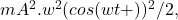## Three different experiments are conducted that pertain to the oscillatory motion of a pendulum. For each experiment, the length of the pend

Question

Three different experiments are conducted that pertain to the oscillatory motion of a pendulum. For each experiment, the length of the pendulum and the mass of the pendulum are indicated in all experiments, the pendulum is released from the same angle with respect to the vertical. If the students collect data bout the kinetic energy of the pendulum as a function of time for each experiment, which of the following claims is true? a. The da ta collected from Experiment 1 will be the same as the data collected from Experiment 2. b. The data collected from Experiment 1 will be the same as the data collected from Experiment 3. C. The data collected from Experiment 2 will be the same as the data collected from Experiment 3. d. The data collected from each experiment will be different.

in progress 0
6 months 2021-07-27T03:26:31+00:00 1 Answers 8 views 0

Their is no data provided for the mass and length of pendulum in the picture.but it is very easy to check kinetic energy.KE is mv^2/2, m is the pendulum Bob and v is time dependent ,equation of displacement of SHM is given as x(t)=Asin(wt+∆) where ∆ is the phase angle now v=dX/dt _v=Awcos(wt+∆) and KE=